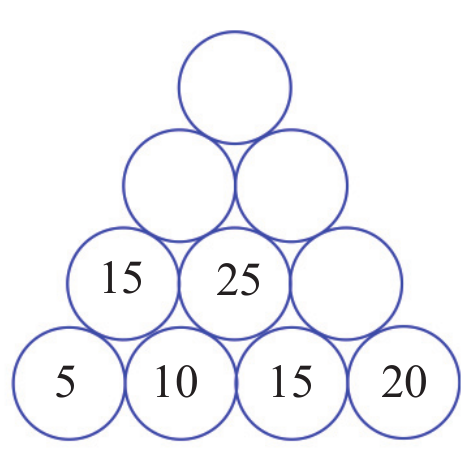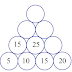## RELATED POSTS1. 5 + 10 = 15
2. 10 + 15 = 25
3. 15 + 20 = 35
4. 15 + 25 = 40
5. 25 + 35 = 60
6. 40 + 60 = 1001. 1 + 3 = 4
2. 3 + 5 = 8
3. 5 + 7 = 12
4. 7 + 9 = 16
5. 4 + 8 = 12
6. 8 + 12 = 20
7. 12 + 16 = 28
8. 12 + 20 = 32
9. 20 + 28 = 48
10. 32 + 48 = 80